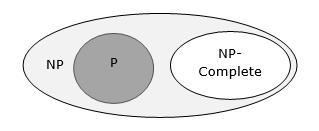• Design and Analysis of Algorithms
• Home

NP Hard and NP-Complete Classes

A problem is in the class NPC if it is in NP and is as hard as any problem in NP. A problem is NP-hard if all problems in NP are polynomial time reducible to it, even though it may not be in NP itself.If a polynomial time algorithm exists for any of these problems, all problems in NP would be polynomial time solvable. These problems are called NP-complete. The phenomenon of NP-completeness is important for both theoretical and practical reasons.

Definition of NP-Completeness

A language B is NP-complete if it satisfies two conditions

• B is in NP

• Every A in NP is polynomial time reducible to B.

If a language satisfies the second property, but not necessarily the first one, the language B is known as NP-Hard. Informally, a search problem B is NP-Hard if there exists some NP-Complete problem A that Turing reduces to B.

The problem in NP-Hard cannot be solved in polynomial time, until P = NP. If a problem is proved to be NPC, there is no need to waste time on trying to find an efficient algorithm for it. Instead, we can focus on design approximation algorithm.

NP-Complete Problems

Following are some NP-Complete problems, for which no polynomial time algorithm is known.

• Determining whether a graph has a Hamiltonian cycle
• Determining whether a Boolean formula is satisfiable, etc.

NP-Hard Problems

The following problems are NP-Hard

• The circuit-satisfiability problem
• Set Cover
• Vertex Cover
• Travelling Salesman Problem

In this context, now we will discuss TSP is NP-Complete

TSP is NP-Complete

The traveling salesman problem consists of a salesman and a set of cities. The salesman has to visit each one of the cities starting from a certain one and returning to the same city. The challenge of the problem is that the traveling salesman wants to minimize the total length of the trip

Proof

To prove TSP is NP-Complete, first we have to prove that TSP belongs to NP. In TSP, we find a tour and check that the tour contains each vertex once. Then the total cost of the edges of the tour is calculated. Finally, we check if the cost is minimum. This can be completed in polynomial time. Thus TSP belongs to NP.

Secondly, we have to prove that TSP is NP-hard. To prove this, one way is to show that Hamiltonian cycle ≤p TSP (as we know that the Hamiltonian cycle problem is NPcomplete).

Assume G = (V, E) to be an instance of Hamiltonian cycle.

Hence, an instance of TSP is constructed. We create the complete graph G' = (V, E'), where

$$E^{'}=\lbrace(i, j)\colon i, j \in V \:\:and\:i\neq j$$

Thus, the cost function is defined as follows −

$$t(i,j)=\begin{cases}0 & if\: (i, j)\: \in E\\1 & otherwise\end{cases}$$

Now, suppose that a Hamiltonian cycle h exists in G. It is clear that the cost of each edge in h is 0 in G' as each edge belongs to E. Therefore, h has a cost of 0 in G'. Thus, if graph G has a Hamiltonian cycle, then graph G' has a tour of 0 cost.

Conversely, we assume that G' has a tour h' of cost at most 0. The cost of edges in E' are 0 and 1 by definition. Hence, each edge must have a cost of 0 as the cost of h' is 0. We therefore conclude that h' contains only edges in E.

We have thus proven that G has a Hamiltonian cycle, if and only if G' has a tour of cost at most 0. TSP is NP-complete.

Useful Video Courses

Video

Compiler Design Online Training

102 Lectures 10 hours

Video

Sequential Circuit Design

30 Lectures 3 hours

Video

Design Patterns Online Training

31 Lectures 4 hours

Video

InDesign Online Training

43 Lectures 1.5 hours

Video

Canva: Become a Graphic Designer

7 Lectures 1 hours

Video

The Ultimate Canva Graphic Design Course

54 Lectures 4 hours